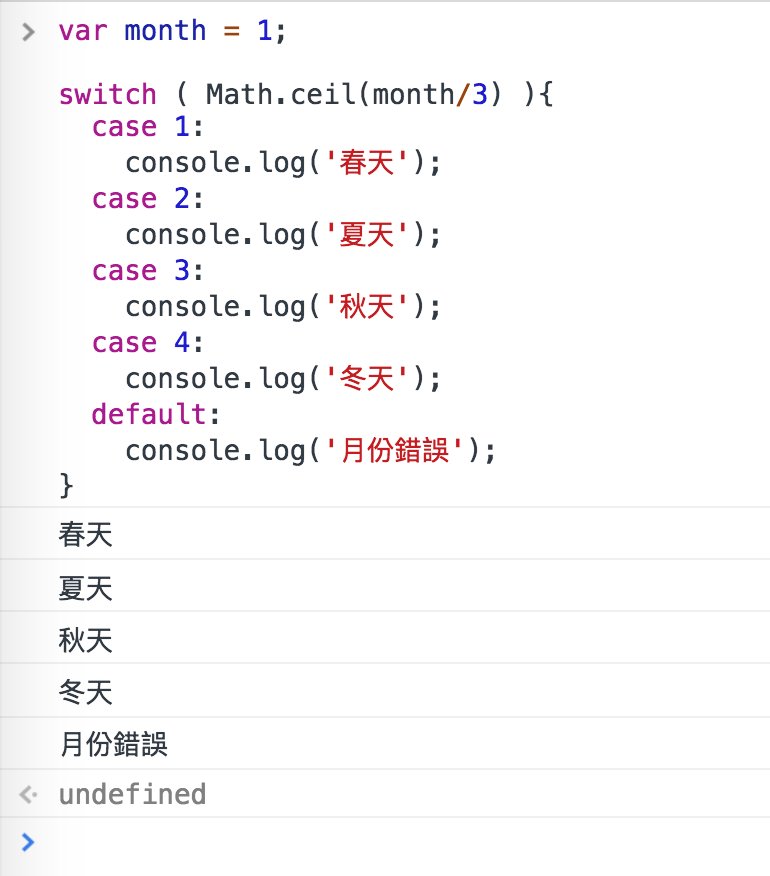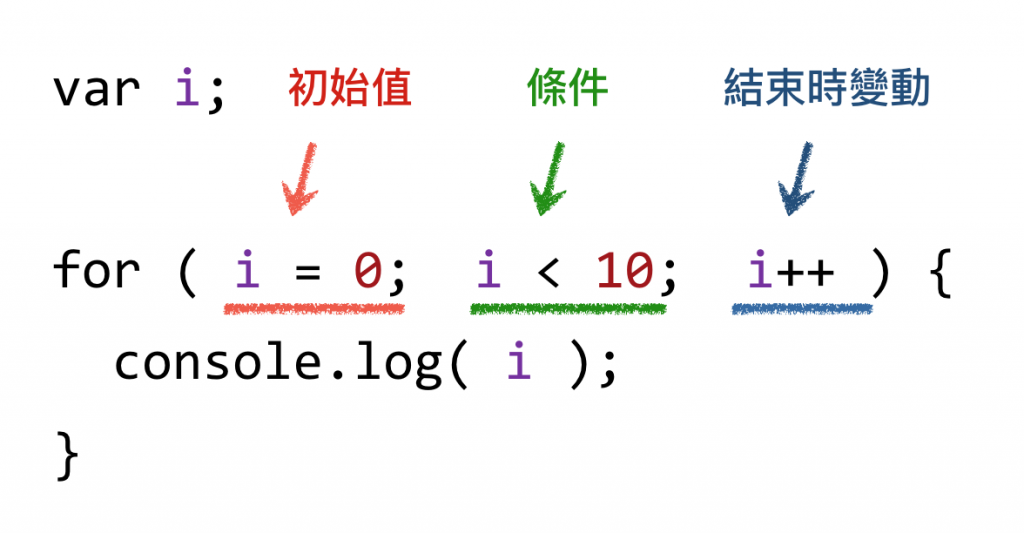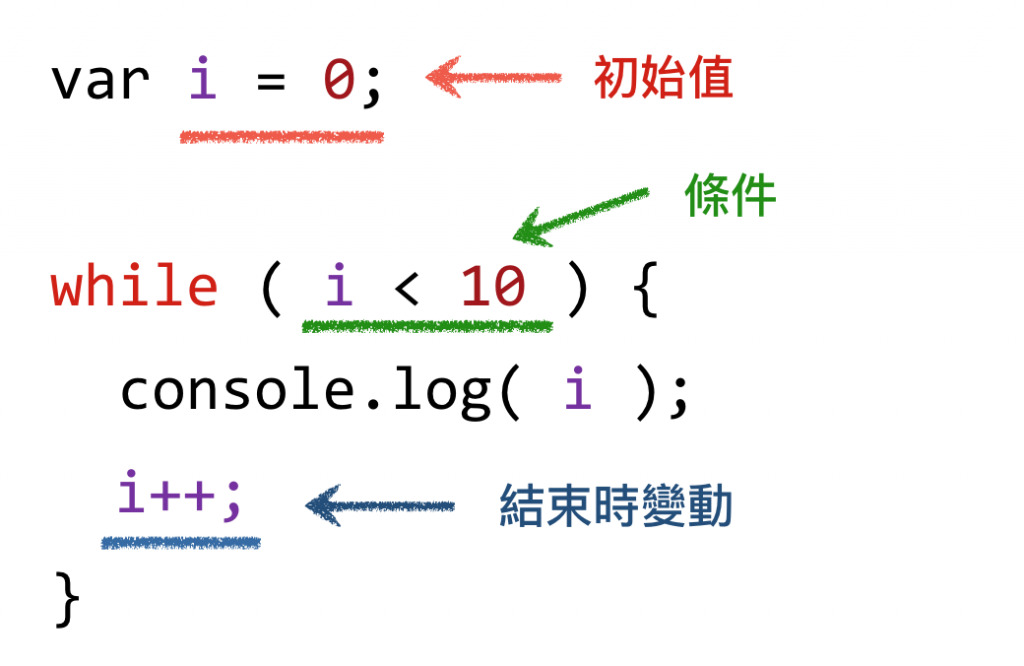#2018 iT 邦幫忙鐵人賽DAY 9
6
Modern Web

## 重新認識 JavaScript: Day 09 流程判斷與迴圈

JavaScript 用來控制流程的「條件語法」指的是，當指定的條件為 `true` 時，就會執行後續所指定的指令。 而 JavaScript 的條件語法有兩種︰ `if...else``switch`

## 條件語法 (1): `if...else`

``````if ( 條件式 ){
// 某條件成立會執行這個 { } 區塊中的內容
// ...
}
else{
// 否則執行 else 這個 { } 區塊中的內容
// else 可以有也可以沒有
}
``````

「JavaScript 所有資料都可以透過 `ToBoolean` 轉換成 `true``false`

``````if ( 條件式 ){
// 某條件成立會執行這個 { } 區塊中的內容
// ...
}
else if( 條件式2 ){
// ...
}
else if( 條件式3 ){
// ...
}
else if( 條件式4 ){
// ...
}
else{
// 若上面所有條件判斷都是 false ，才會執行 else 這個 { } 區塊中的內容

}
``````

`else if` 基本上沒有數量的限制，但為了程式的可讀性與維護性，還是盡量減少 `if...else` 的條件數量喔。

``````// 下班買十顆包子回家，如果看到賣西瓜的，就買一個。

if( 看到賣西瓜的 ) {
}
``````

## 條件語法 (2): `switch`

``````if ( 條件式 ){
// 某條件成立會執行這個 { } 區塊中的內容
// ...
}
else if( 條件式2 ){
// ...
}
else if( 條件式3 ){
// ...
}
else if( 條件式4 ){
// ...
}
else{
// 若上面所有條件判斷都是 false ，才會執行 else 這個 { } 區塊中的內容
// ...
}
``````

``````var month = 12;

// 註：Math.ceil(n) 代表將 n 數值無條件進位

if( Math.ceil( month/3 ) === 1 ){
console.log('春天');
}
else if( Math.ceil( month/3 ) === 2 ){
console.log('夏天');
}
else if( Math.ceil( month/3 ) === 3 ){
console.log('秋天');
}
else if( Math.ceil( month/3 ) === 4 ){
console.log('冬天');
}
else{
console.log('月份錯誤');
}
``````

``````var month = 12;

switch ( Math.ceil(month/3) ){
case 1:
console.log('春天');
break;
case 2:
console.log('夏天');
break;
case 3:
console.log('秋天');
break;
case 4:
console.log('冬天');
break;
default:
console.log('月份錯誤');
break;
}
``````

`default` 的區塊就是當上面所有 `case` 都不成立的時候會執行。

``````var month = 1;

switch ( Math.ceil(month/3) ){
case 1:
console.log('春天');
case 2:
console.log('夏天');
case 3:
console.log('秋天');
case 4:
console.log('冬天');
default:
console.log('月份錯誤');
}
````````````var month = 1;

switch ( month ){
case 1:
case 2:
case 3:
console.log('春天');
break;
case 4:
case 5:
case 6:
console.log('夏天');
break;
case 7:
case 8:
case 9:
console.log('秋天');
break;
case 10:
case 11:
case 12:
console.log('冬天');
break;
default:
console.log('月份錯誤');
}
``````

## 三元運算子: `(條件式) ? A : B`

``````(條件) ? [數值1] : [數值2];
``````

``````var status = (age >= 18) ? '成人' : '小孩';
``````

## 迴圈

``````console.log(1);
console.log(2);
console.log(3);
console.log(4);
console.log(5);
console.log(6);
console.log(7);
console.log(8);
console.log(9);
console.log(10);
``````## `for` 迴圈

``````var i;

for (i = 0; i < 10; i++) {
// 做某件事
}
````````````for (var i = 0; i < 10; i++) {
// 做某件事
}
``````

``````for (var i = 1; i <= 10; i++) {
console.log(i);
}
``````

## `while` 迴圈

``````var i = 1;

while ( i <= 10 ){
console.log( i );
i++;
}
```````for` 相比，兩者在執行時的原理其實大同小異。

``````// i 不管怎麼樣都會大於等於 0

for (var i = 0; i >= 0; i++) {
console.log(i);
}
``````

``````var i = 1;

// 結束迴圈時， i 的值仍然是 1
while ( i <= 10 ){
console.log( i );
}
``````

## `break` 與 `continue`

• `break` 會直接跳離迴圈
• `continue` 會跳過一次，然後繼續下一次迴圈。

``````for (var i = 1; i <= 10; i++) {

// i 能被 3 整除表示 i 是 3 的倍數，遇到 continue 就會跳過這次
if( i % 3 === 0){
continue;
}

console.log(i);
}
``````

``````// 假設陣列是這樣包含一堆 0 與若干其他數字
var arr = [0,0,0,0,7,0,9,0,4,8,0];

for(var i = 0; i < arr.length; i++ ) {

if( arr[i] !== 0 ){
// 找到那個不是 0 的數字，印出後退出迴圈
console.log( arr[i] );
break;
}
}

``````

## `for` 與 `while` 兩者的差異點

• `for` 迴圈的使用情境，大多是用在迴圈執行次數「明確」的狀態
• `while` 迴圈則剛好相反，當迴圈執行次數「不確定」的時候更適合。

`while` 迴圈的話，你可以這樣寫：

``````var lottery = [];
var n;

// 直到陣列 lottery 選滿 6 球
while(lottery.length < 6) {

// 取一隨機 1 ~ 49 數字
n = Math.floor( Math.random() * 49 ) + 1;

// 如果選出來的 n 不存在，就放入陣列
if( lottery.indexOf( n ) === -1 ){
lottery.push( n );
}
}
``````

### 3 則留言

1
``````  if( lottery.indexOf( n ) !== -1 ){
lottery.push( n );
}
``````

1

``````var buy = "十顆包子";

if( 看到賣西瓜的 ) {
}
``````
1

for (var i = 1; i <= 10; i++) {

for ( i = 1; i <= 10; i++) {

noway iT邦新手 5 級‧ 2019-08-28 20:54:47 檢舉

noway iT邦新手 5 級‧ 2019-08-28 21:04:11 檢舉

var x=1 ; //非全域
y=2; //全域
}

?謝謝!

``````function func(){
var x = 1;
y = 2;
}

func();
``````